Vous devez avoir un compte Developpez.com et être connecté pour pouvoir participer aux discussions.

##### Identifiez-vous
 Identifiant Mot de passe Mot de passe oublié ?
##### Créer un compte

Vous n'avez pas encore de compte Developpez.com ? L'inscription est gratuite et ne vous prendra que quelques instants !Developpez.com

Python

Choisissez la catégorie, puis la rubrique :

17.7. plural.py, stage 6

## 17.7. plural.py, stage 6

### Example 17.17. plural6.py

```
import re

def rules(language):
for line in file('rules.%s' % language):
pattern, search, replace = line.split()
yield lambda word: re.search(pattern, word) and re.sub(search, replace, word)

def plural(noun, language='en'):
for applyRule in rules(language):
result = applyRule(noun)
if result: return result
```

This uses a technique called generators, which I'm not even going to try to explain until you look at a simpler example first.

### Example 17.18. Introducing generators

```>>> def make_counter(x):
...     print 'entering make_counter'
...     while 1:
...         yield x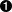...         print 'incrementing x'
...         x = x + 1
...
>>> counter = make_counter(2)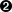>>> counter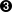<generator object at 0x001C9C10>
>>> counter.next()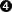entering make_counter
2
>>> counter.next()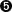incrementing x
3
>>> counter.next()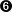incrementing x
4
```The presence of the yield keyword in make_counter means that this is not a normal function. It is a special kind of function which generates values one at a time. You can think of it as a resumable function. Calling it will return a generator that can be used to generate successive values of x.To create an instance of the make_counter generator, just call it like any other function. Note that this does not actually execute the function code. You can tell this because the first line of make_counter is a print statement, but nothing has been printed yet.The make_counter function returns a generator object.The first time you call the next() method on the generator object, it executes the code in make_counter up to the first yield statement, and then returns the value that was yielded. In this case, that will be 2, because you originally created the generator by calling make_counter(2).Repeatedly calling next() on the generator object resumes where you left off and continues until you hit the next yield statement. The next line of code waiting to be executed is the print statement that prints incrementing x, and then after that the x = x + 1 statement that actually increments it. Then you loop through the while loop again, and the first thing you do is yield x, which returns the current value of x (now 3).The second time you call counter.next(), you do all the same things again, but this time x is now 4. And so forth. Since make_counter sets up an infinite loop, you could theoretically do this forever, and it would just keep incrementing x and spitting out values. But let's look at more productive uses of generators instead.

### Example 17.19. Using generators instead of recursion

```
def fibonacci(max):
a, b = 0, 1while a < max:
yield aa, b = b, a+b```The Fibonacci sequence is a sequence of numbers where each number is the sum of the two numbers before it. It starts with 0 and 1, goes up slowly at first, then more and more rapidly. To start the sequence, you need two variables: a starts at 0, and b starts at 1.a is the current number in the sequence, so yield it.b is the next number in the sequence, so assign that to a, but also calculate the next value (a+b) and assign that to b for later use. Note that this happens in parallel; if a is 3 and b is 5, then a, b = b, a+b will set a to 5 (the previous value of b) and b to 8 (the sum of the previous values of a and b).

So you have a function that spits out successive Fibonacci numbers. Sure, you could do that with recursion, but this way is easier to read. Also, it works well with for loops.

### Example 17.20. Generators in for loops

```>>> for n in fibonacci(1000):...     print n,0 1 1 2 3 5 8 13 21 34 55 89 144 233 377 610 987
```You can use a generator like fibonacci in a for loop directly. The for loop will create the generator object and successively call the next() method to get values to assign to the for loop index variable (n).Each time through the for loop, n gets a new value from the yield statement in fibonacci, and all you do is print it out. Once fibonacci runs out of numbers (a gets bigger than max, which in this case is 1000), then the for loop exits gracefully.

OK, let's go back to the plural function and see how you're using this.

### Example 17.21. Generators that generate dynamic functions

```
def rules(language):
for line in file('rules.%s' % language):pattern, search, replace = line.split()yield lambda word: re.search(pattern, word) and re.sub(search, replace, word)def plural(noun, language='en'):
for applyRule in rules(language):result = applyRule(noun)
if result: return result
```for line in file(...) is a common idiom for reading lines from a file, one line at a time. It works because file actually returns a generator whose next() method returns the next line of the file. That is so insanely cool, I wet myself just thinking about it.No magic here. Remember that the lines of the rules file have three values separated by whitespace, so line.split() returns a tuple of 3 values, and you assign those values to 3 local variables.And then you yield. What do you yield? A function, built dynamically with lambda, that is actually a closure (it uses the local variables pattern, search, and replace as constants). In other words, rules is a generator that spits out rule functions.Since rules is a generator, you can use it directly in a for loop. The first time through the for loop, you will call the rules function, which will open the rules file, read the first line out of it, dynamically build a function that matches and applies the first rule defined in the rules file, and yields the dynamically built function. The second time through the for loop, you will pick up where you left off in rules (which was in the middle of the for line in file(...) loop), read the second line of the rules file, dynamically build another function that matches and applies the second rule defined in the rules file, and yields it. And so forth.

What have you gained over stage 5? In stage 5, you read the entire rules file and built a list of all the possible rules before you even tried the first one. Now with generators, you can do everything lazily: you open the first and read the first rule and create a function to try it, but if that works you don't ever read the rest of the file or create any other functions.